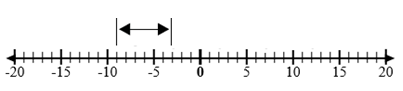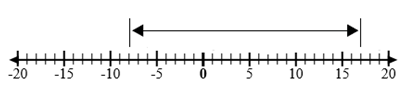# Computing the distance between two integers on a number line

#### Operations Management Online Training

15 Lectures 1 hours

#### Customer Service Excellence - from customers to operations

69 Lectures 6 hours

#### Management Series- Know how to develop a Budget for Business Operations

4 Lectures 33 mins

A simple way to calculate the distance between numbers on a number line is to count every number between them. A faster way is to find the distance by taking the absolute value of the difference of those numbers.

Absolute value of a number on a number line is its distance from 0 on the number line

For example, the absolute values of 4 and -4, or |4| and |-4|, are both 4.

This method of finding distance on number line works for numbers both close together and far apart.

For example, the distance between 4 and −4 on the number line given by the |4 – (−4) | = |4 + 4 | = 8 units

Use the number line to find the distance between the following integers:

−9 and −3

### Solution

Step 1:

On the number lineStep 2:

Distance between the integers

|−3 −(−9) | = |−3 + 9| = |6| = 6 units

Use the number line to find the distance between the following integers:

17 and −8

### Solution

Step 1:

On the number lineStep 2:

Distance between the integers

|17 −(−8) | = |17 + 8 | = |25| = 25 units